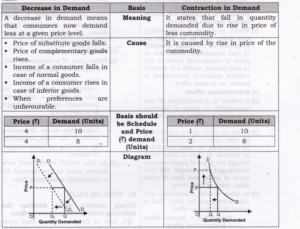# Chapter 3 – Demand Questions and Answers: NCERT Solutions for Class 12 Micro Economics

Class 12 Introduction to Economics NCERT book solutions for Chapter 3 - Demand Questions and Answers.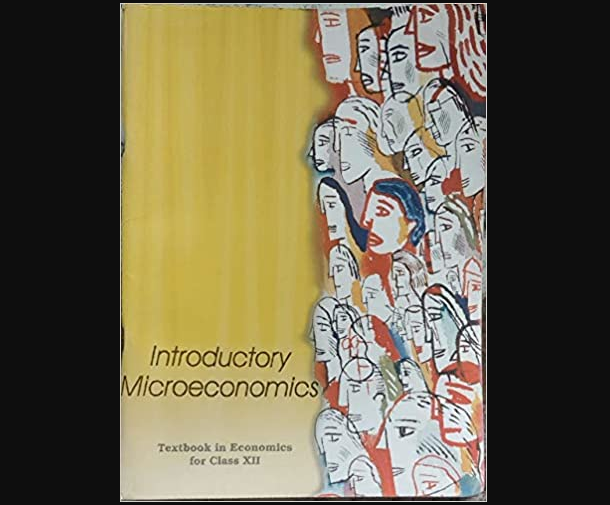## Question 1. Suppose there are two consumers in the market for a good and their demand functions are as follows: D1(p)=20−P for any price than less 20, and D1(p)=0 at any price greter than or equal to 20. D2(p)=30−2P for any price less than 15 and D2(p)=0 at any price greater than or equal to 15. Find out the market demand function.

### Answer: It can be seen from the given demand functions that Consumer 1 do not want to demand the goods for any price greater than or equal Rs.20 and consumer 2 do not want to demand the goods for any price greater than Rs 15. Hence, the market demand function will be,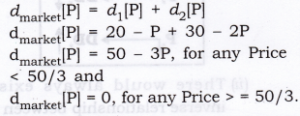## Question 2. Suppose there are 20 consumers for a good and they have identical demand function?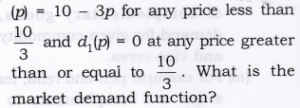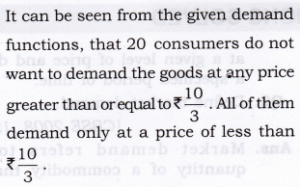## Question 3.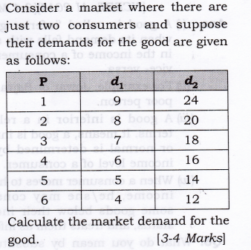## Question 6. What do you mean by substitutes? Give examples of two goods which are substitutes of each other.

### Answer: 1. Substitute goods are those goods which can be used in place of another goods and give the same satisfaction to a consumer.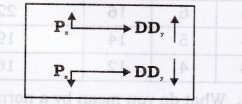## Question 7. What do you mean by complements? Give examples of two goods which are complements of each other.

### Answer: 1. Complementary goods are those which are useless in the absence of other goods and which are demanded jointly.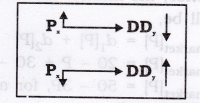## Question 5. Differentiate between Normal Goods and Inferior Goods.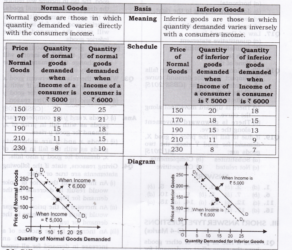## Question 6. Differentiate between substitute goods and complementary goods.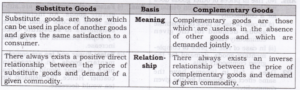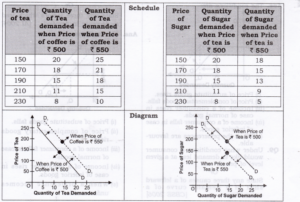## Question 7. Explain law of demand with the help of a demand schedule.

### Answer: 1. It states that price of the commodity and quantity demanded are inversely related to each other, when other factors remain constant. It means, quantity demanded of the commodity rises due to fall in price of the commodity and vice-versa. 2. Ceteris Paribus means: (a) Price of Related commodity remains constant. (b) Income of a consumer remains constant. (c) Taste and preferences of a household remains constant.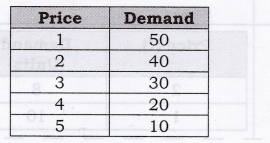## Question 8. Under what conditions a consumer would like to demand more at a given level of price? Or State any three causes of a rightward shift of a demand curve of a commodity. State any three factors which lead to increase in demand.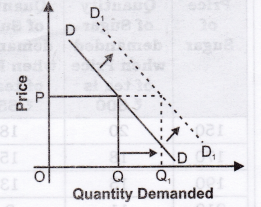## Question 9. Under what conditions a consumer would like to demand less at a given level of price? Or State any three causes of a leftward shift of a demand curve of a commodity. State any three factors which lead to decrease in demand.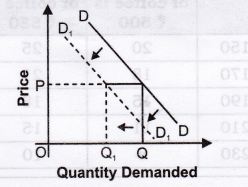## Question 10. Differentiate between increase in demand and expansion in demand (increase in quantity demanded).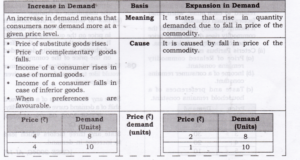## Question 11. Differentiate between decrease in demand and contraction in demand (decrease in quantity demanded).